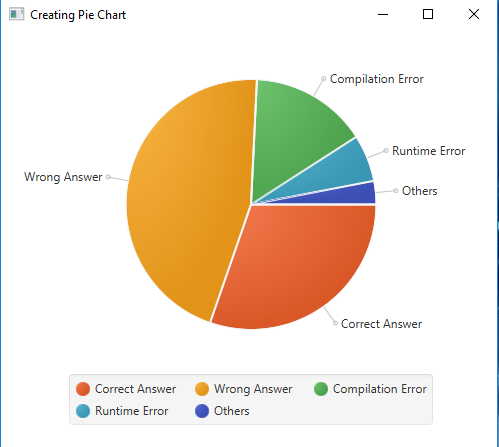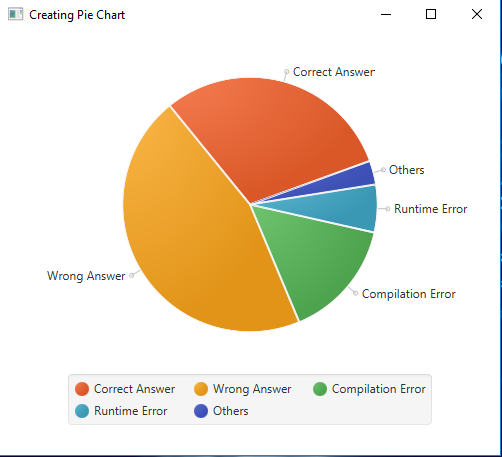# JavaFX | PieChart Class

PieChart class is a part of JavaFX. PieChart class is used to create a pie chart. The chart content is populated by pie slices based on data set on the PieChart.The layout of the pie chart is set to clockwise by default. PieChart inherits Chart class.

Constructor of the class are:

1. PieChart(): Creates an empty instance of pie chart.
2. PieChart(ObservableList data): Creates an instance of pie chart with given data.

Commonly Used Methods:

Methods Explanation
getData() returns the data of the pie chart
getLabelLineLength() returns the label length of the pie chart
getLabelsVisible() Indicates whether pie slice labels are drawn or not
getStartAngle() returns the start angle of the pie chart
isClockwise() returns whether the pie chart is clockwise or not
setClockwise(boolean v) sets the pie chart orientation to clockwise is true value is passed
setData(ObservableList data) Sets the value of the property data.
setLabelLineLength(double v) sets the label line length of pie chart .
setLabelsVisible(boolean v) Sets the value of the property labelsVisible.
setStartAngle(double v) sets the start angle of the the pie chart

Below programs will illustrate the use of the PieChart class:

1. Java program to create a pie chart with some specified data: This program creates a PieChart. A PieChart.Data will be created that will be added to the pie chart as an observable list.T he PieChart will be created inside a scene, which in turn will be hosted inside a stage. The function setTitle() is used to provide title to the stage. Then a Group is created, and the pie chart is attached. The group is attached to the scene. Finally, the show() method is called to display the final results.

 `// Java program to create a pie chart  ` `// with some specified data ` `import` `javafx.application.Application; ` `import` `javafx.scene.Scene; ` `import` `javafx.scene.chart.PieChart; ` `import` `javafx.scene.layout.*; ` `import` `javafx.event.ActionEvent; ` `import` `javafx.scene.AmbientLight; ` `import` `javafx.scene.shape.Sphere; ` `import` `javafx.scene.control.*; ` `import` `javafx.stage.Stage; ` `import` `javafx.scene.Group; ` `import` `javafx.scene.PerspectiveCamera; ` `import` `javafx.scene.paint.Color; ` `import` `javafx.event.ActionEvent; ` `import` `javafx.event.EventHandler; ` `import` `javafx.collections.FXCollections; ` `  `  `public` `class` `pie_chart_1 ``extends` `Application { ` `  `  `    ``// launch the application ` `    ``public` `void` `start(Stage stage) ` `    ``{ ` `        ``// set title for the stage ` `        ``stage.setTitle(``"Creating Pie Chart"``); ` `  `  `        ``// piechart data ` `        ``PieChart.Data data[] = ``new` `PieChart.Data[``5``]; ` `  `  `        ``// string and integer data ` `        ``String status[] = {``"Correct Answer"``, ``"Wrong Answer"``,  ` `                           ``"Compilation Error"``, ``"Runtime Error"``, ` `                           ``"Others"` `}; ` `                             `  `        ``int` `values[] = {``20``, ``30``, ``10``, ``4``, ``2``}; ` `  `  `        ``for` `(``int` `i = ``0``; i < ``5``; i++) { ` `            ``data[i] = ``new` `PieChart.Data(status[i], values[i]); ` `        ``} ` `  `  `        ``// create a pie chart ` `        ``PieChart pie_chart = ``new` `                ``PieChart(FXCollections.observableArrayList(data)); ` `  `  `        ``// create a Group ` `        ``Group group = ``new` `Group(pie_chart); ` `  `  `        ``// create a scene ` `        ``Scene scene = ``new` `Scene(group, ``500``, ``300``); ` `  `  `        ``// set the scene ` `        ``stage.setScene(scene); ` `  `  `        ``stage.show(); ` `    ``} ` `  `  `  `  `    ``// Main Method ` `    ``public` `static` `void` `main(String args[]) ` `    ``{ ` `          `  `        ``// launch the application ` `        ``launch(args); ` `    ``} ` `} `

Output:2. Java program to create a pie chart with some specified data, with visible labels and a defined start angle, and ordered in anticlockwise direction: This program creates a PieChart. A PieChart.Data will be created that will be added to the pie chart as an observable list. The PieChart will be created inside a scene, which in turn will be hosted inside a stage. The function setTitle() is used to provide title to the stage. Then a Group is created, and the pie chart is attached. The group is attached to the scene. Finally, the show() method is called to display the final results.we will set label line length of pie chart using setLabelLineLength() function, we will set the start angle and the clockwise orientation using setStartAngle() and setClockwise() function respectively . we can make the labels visible using setLabelsVisible() function.

 `// Java program to create a pie chart with ` `// some specified data, with visible labels ` `// and a defined start angle, and ordered  ` `// in anticlockwise direction ` `import` `javafx.application.Application; ` `import` `javafx.scene.Scene; ` `import` `javafx.scene.chart.PieChart; ` `import` `javafx.scene.layout.*; ` `import` `javafx.event.ActionEvent; ` `import` `javafx.scene.AmbientLight; ` `import` `javafx.scene.shape.Sphere; ` `import` `javafx.scene.control.*; ` `import` `javafx.stage.Stage; ` `import` `javafx.scene.Group; ` `import` `javafx.scene.PerspectiveCamera; ` `import` `javafx.scene.paint.Color; ` `import` `javafx.event.ActionEvent; ` `import` `javafx.event.EventHandler; ` `import` `javafx.collections.FXCollections; ` `  `  `public` `class` `pie_chart_2 ``extends` `Application { ` `  `  `    ``// launch the application ` `    ``public` `void` `start(Stage stage) ` `    ``{ ` `        ``// set title for the stage ` `        ``stage.setTitle(``"Creating Pie Chart"``); ` `  `  `        ``// piechart data ` `        ``PieChart.Data data[] = ``new` `PieChart.Data[``5``]; ` `  `  `        ``// string and integer data ` `        ``String status[] = {``"Correct Answer"``, ``"Wrong Answer"``,  ` `                           ``"Compilation Error"``, ``"Runtime Error"``,  ` `                           ``"Others"``}; ` `                             `  `        ``int` `values[] = {``20``, ``30``, ``10``, ``4``, ``2``}; ` `  `  `        ``for` `(``int` `i = ``0``; i < ``5``; i++) { ` `            ``data[i] = ``new` `PieChart.Data(status[i], values[i]); ` `        ``} ` `  `  `        ``// create a pie chart ` `        ``PieChart pie_chart = ``new` `                ``PieChart(FXCollections.observableArrayList(data)); ` `  `  `        ``// set line length of label ` `        ``pie_chart.setLabelLineLength(``10``.0f); ` `  `  `        ``// make labels visible ` `        ``pie_chart.setLabelsVisible(``true``); ` `  `  `        ``// set start angle ` `        ``pie_chart.setStartAngle(``20``.0f); ` `  `  `        ``// set anticlockwise ` `        ``pie_chart.setClockwise(``false``); ` `  `  `        ``// create a Group ` `        ``Group group = ``new` `Group(pie_chart); ` `  `  `        ``// create a scene ` `        ``Scene scene = ``new` `Scene(group, ``500``, ``500``); ` `  `  `        ``// set the scene ` `        ``stage.setScene(scene); ` `  `  `        ``stage.show(); ` `    ``} ` `  `  `    ``// Main Method ` `    ``public` `static` `void` `main(String args[]) ` `    ``{ ` `          `  `        ``// launch the application ` `        ``launch(args); ` `    ``} ` `} `

Output:Note: The above programs might not run in an online IDE. Please use an offline compiler.

Attention reader! Don’t stop learning now. Get hold of all the important DSA concepts with the DSA Self Paced Course at a student-friendly price and become industry ready.

My Personal Notes arrow_drop_upCheck out this Author's contributed articles.

If you like GeeksforGeeks and would like to contribute, you can also write an article using contribute.geeksforgeeks.org or mail your article to contribute@geeksforgeeks.org. See your article appearing on the GeeksforGeeks main page and help other Geeks.

Please Improve this article if you find anything incorrect by clicking on the "Improve Article" button below.

Article Tags :
Practice Tags :

Be the First to upvote.

Please write to us at contribute@geeksforgeeks.org to report any issue with the above content.